# Communications - Online Test

Q1. A load of 50 Ω is connected in shunt in a 2-wire transmission line of Z0 = 50Ω as shown in the figure. The 2-port scattering parameter matrix (s-matrix) of the shunt element is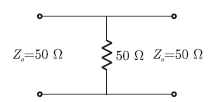Explaination / Solution: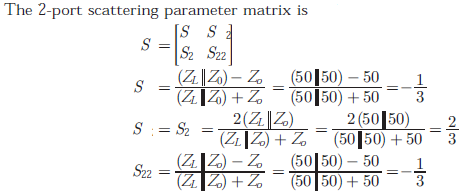Q2. Any physical quantity like temperature can be converted to
Explaination / Solution:

An electrical transducer is a device which is capable of converting the physical quantity into a proportional electrical quantity such as voltage or electric current. Hence it converts any quantity to be measured into usable electrical signal. This physical quantity which is to be measured can be pressure, level, temperature, displacement etc. The output which is obtained from the transducer is in the electrical form and is equivalent to the measured quantity. For example, a temprature transducer will convert temperature to an equivalent electrical potential. This output signal can be used to control the physical quantity or display it.moreover this is of two type Digital or Analog electrical signals.

Q3. The parallel branches of a 2-wire transmission line re terminated in 100Ω and 200Ω resistors as shown in the figure. The characteristic impedance of the line is Z0 = Ω and each section has a length of λ/4. The voltage reflection coefficient Γ at the input is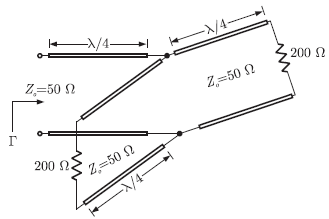Explaination / Solution: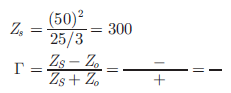Q4. In Analog type signals
Explaination / Solution:

A signal in which current or voltage varies with time is called analog signal.A vibrating fork produces such a sound signal of single frequency.Every speech or music is a complex signal formed by the superposition of several sinusoidal signals of different amplitudes and frequencies.

Q5. A λ/2 dipole is kept horizontally at a height of λ0/2 above a perfectly conducting infinite ground plane. The radiation pattern in the lane of the dipole (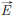plane) looks approximately as
Explaination / Solution: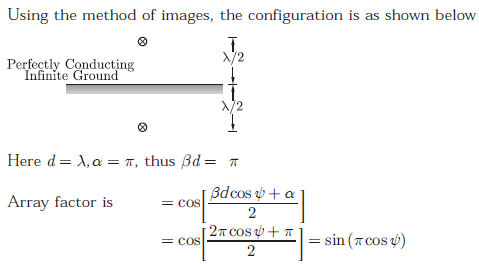Q6. In Digital type signals, signals can take
Explaination / Solution:
No Explaination.

Q7. A right circularly polarized (RCP) plane wave is incident at an angle 600 to the normal, on an air-dielectric interface. If the reflected wave is linearly polarized, the relative dielectric constant ξr is.Explaination / Solution:
No Explaination.

Q8. Noise in electronic communication refers to
Explaination / Solution:

In communication systems, noise is an error or undesired random disturbance of a useful information signal. The noise is a summation of unwanted or disturbing energy from natural and sometimes man-made sources.Consequently,a distored or corrupted version of the transmitted signal reaches the receiver.

Q9. Two 4-array signal constellations are shown. It is given that φ1 and φ2 constitute an orthonormal basis for the two constellation. Assume that the four symbols in both the constellations are equiprobable. Let N0/2 denote the power spectral density of white Gaussian noise.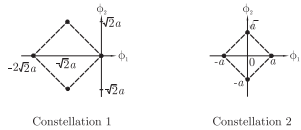The if ratio or the average energy of Constellation 1 to the average energy of Constellation 2 is
Explaination / Solution:Q10. Transmitter is a device that
Explaination / Solution:

A transmitter is a set up that transmits the message to the receiving end through a communication channel.its main function is to modify the message signal in a form suitable for transmission over the channel and to transmit it.The transmitter is able to generate a radio frequency alternating current that is then applied to the antenna, which, in turn, radiates this as radio waves. There are many types of transmitters depending on the standard being used and the type of device; for example, many modern devices that have communication capabilities have transmitters such as Wi-Fi, Bluetooth, NFC and cellular.